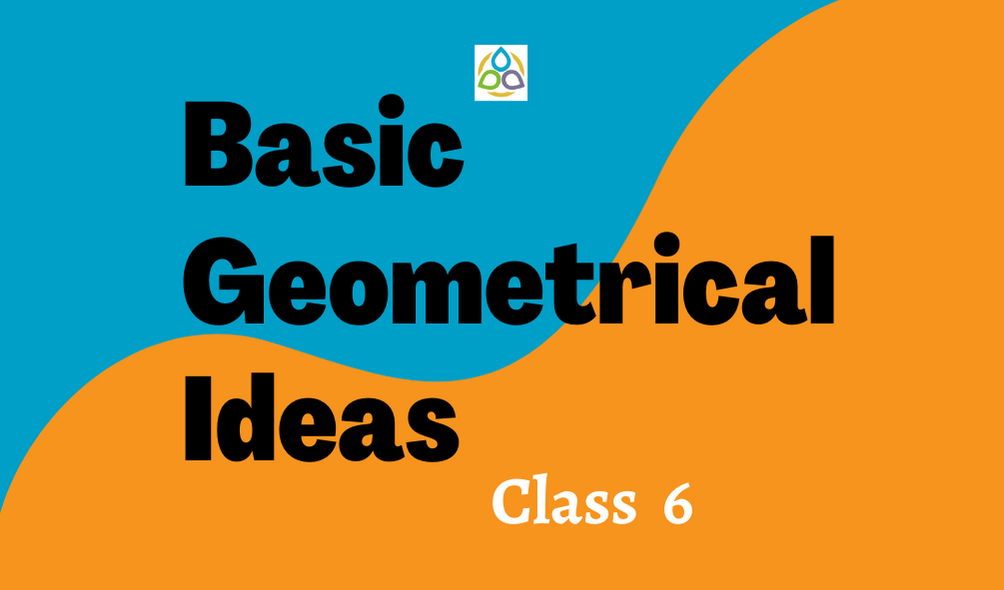# Basic Geometrical Ideas

75. A point determines a

• (A) Space
• (B) Location
• (C) Shortest distance
• (D) Area

74. The shortest distance between two points corresponds to

• (A) Line
• (B) Line segment
• (C) Ray
• (D) Curve

73. Two distinct  lines  in  a  plane  that  cross  at  a  point  are  called

• (A) Intersecting lines
• (B) Parallel lines
• (C) Non-intersecting lines
• (D) Non-parallel lines

72. Two distinct lines in a plane that do not meet are called

• (A) Intersecting lines
• (B) Parallel lines
• (C) Non-intersecting lines
• (D) Non-parallel lines

71. How many lines can pass through one given point?

• (A) Zero
• (B) One
• (C) Two
• (D) Infinite

70. How many lines can pass through two given points?

• (A) Zero
• (B) One
• (C) Two
• (D) Infinite

69. A simple closed curve made of line segments only is called a

• (A) Polygon
• (B) Line
• (C) Circle
• (D) None of these

68. A polygon of three sides is called a

• (A) Triangle
• (B) Square
• (C) Line
• (D) Point

67. A polygon of four sides is called a

• (A) Triangle
• (C) Line
• (D) Point

66. Regular polygon means

• (A) A polygon with all sides equal
• (B) A polygon with all angles equal
• (C) A polygon with all sides and all angles equal
• (D) A polygon with all unequal sides and unequal angles

65. A figure, every point of which is equidistant from a fixed point is called a

• (A) Point
• (B) Square
• (C) Circle
• (D) Pentagon

64. A figure, every point of which is equidistant from a fixed point is called a circle. The fixed point is known as

• (B) Diameter
• (C) Centre
• (D) Circumference

63. A figure, every point of which is equidistant from a fixed point is called a circle. The equal distance is called its

• (B) Diameter
• (C) Centre
• (D) Circumference

62. The distance around the circle is known as